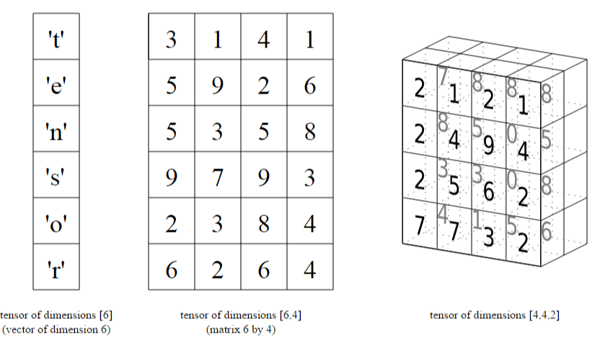Related Tags

tensor

# What are Tensors?

Talha Ashar

Tensors are multi-dimensional arrays that store numerical data. Tensors can be thought of as a mathematical entity, similar to a generalized matrix, that interacts with other entities in a structure.

## Types of tensors

The table below shows the different types of tensors and the names conventionally associated with each type:

Tensor Types

Unlike a matrix, the numerical data stored in the tensor shifts given a transformation in one of the entities it interacts with. As such, tensors always obey certain transformation rules.

Note: In deep learning, a 2-D Array is referred to as a matrix, and arrays with more than $2$ dimensions are called tensors.## Attributes

The main attributes of tensors are as follows:

• Rank: The number of dimensions present within the tensor. For example, a $2$-dimensional tensor has a rank of $2$, while a $3$-dimensional tensor has a rank of $3$.

• Axis: A specific dimension of a tensor. For example, a $2$-dimensional tensor has $2$ axes.

• Shape: The length of each axis of a tensor. It is possible to reshape a tensor without disturbing any of the elements it contains.

Let’s take a look at each of these attributes with the help of an example. First, consider the tensor shown below:

t = [[1,2,3], [4,5,6], [7,8,9]]


The tensor t has $2$ dimensions, so it has a rank of $2$ and $2$ possible axes.

The elements along the first axis ([1,2,3], [4,5,6], and [7,8,9]) represent arrays, whereas each value within these arrays (the second axis) represents the data.

Since the tensor t has $3$ rows and $3$ columns, the shape will be [3, 3].

## Applications

Tensors provide great flexibility in their dimensions and shape, and therefore they are commonly used to store datasets that contain multi-dimensional information.

Some common applications are as follows:

• 3-D Tensors: Used to store time-series data, e.g., medical scans. Each dimension in the tensor may correspond to frequency, time, and channels of signals, respectively. If data for multiple patients is needed, a 4-D tensor may be used, where the extra dimension represents the sample size.

• 4-D Tensors: Used to store JPEG images. Each dimension may correspond to the sample size, height, width, and color depth of the image, respectively.

• 5-D Tensors: Used to store video data. Each dimension may correspond to the sample size, frames, height, width, and color depth, respectively.

## Example

The example below shows how tensors are used in the Pytorch library:

import torch

# initialize tensor
a = torch.tensor([[1,2,3], [4,5,6], [7,8,9]])
b = torch.tensor([[1,2,3], [4,5,6], [7,8,9]], dtype=torch.int32)

# printing tensors
print("Tensor A: ", a)
print("Tensor B: ", b)

# extracting values
c = a
d = a
print("The extracted values are", c, "and", d)

e = c.item()
f = d.item()
print("The extracted values are", e, "and", f)

## Explanation

First, two tensor objects, a and b, are initialized using python lists in lines 4 and 5. For b, the tensor is initialized with a specific data type since the dtype attribute is provided during initialization.

You can extract a particular dimension of the tensor through python indexing. For example, in line 12, the tensor a is indexed for the value in the third column of its second row, i.e., a. This indexing returns a tensor object, which can be converted into a simple numeric data type using the item function, as shown in lines 16 and 17.

Note: A comprehensive guide on tensors and their operations in Pytorch can be found in the Official Guide.

RELATED TAGS

tensor

CONTRIBUTOR

Talha Ashar